# deBruijn

## Mathematical Details

This page explains in detail how the Penrose tiling, and other related quasiperiodic tilings drawn by the deBruijn applet, are constructed from a hypercubic lattice in Rn. It also discusses the generalisation of these methods to higher dimensional tilings, and to other lattices (including the An lattice used by the Tübingen applet).

### Quasiperiodic tilings of the plane from the hypercubic lattice

#### Construction

We define the points of the lattice Zn to be those (x1, …, xn) in Rn where all the xi have integer values. These points comprise the vertices of an infinite number of unit hypercubes packed together to fill Rn, so we will refer to this as the “hypercubic lattice”.

Suppose we choose an affine plane P in Rn parallel to the two-dimensional subspace spanned by the two vectors:

x = (1, –cos(π/n), cos(2π/n), …, (–1)(n–1)cos((n–1)π/n))
y = (0, –sin(π/n), sin(2π/n), …, (–1)(n–1)sin((n–1)π/n))

These are not unit vectors; if we want to normalise them we need to divide by √(n/2).

The projections of the n coordinate axes and their opposites onto P form a symmetrical 2n-pointed star. Note that for odd values of n, P is orthogonal to one of the hypercubic lattice diagonals, (1,1,1,1,…,1), but this is not the case for even n; if it were, the projections of the 2n vectors would overlap each other to form an n-pointed star.

We are interested in identifying squares within the lattice that “approximate” the plane P, in the sense that all four of these squares’ vertices can fit simultaneously within a unit-side-length hypercube whose centre lies somewhere on P and which is oriented parallel to the hypercubes of the lattice itself. Because the lattice has a spacing of 1, and the hypercube whose centre lies on the plane has a side length of 1, if a whole square from the lattice ever lies inside the hypercube then the hypercube’s centre must have the two coordinates corresponding to the square’s edge directions equal to those of the centre of the square itself. In other words, those coordinates will have odd-half-integer values, midway between the integer values of the lattice points.

If we define n families of hyperplanes in Rn by fixing each of the coordinates xi at odd-half-integer values, then the intersections of P with these families of hyperplanes will be sets of parallel lines in P. Wherever two of these lines intersect at a point Q, a unit hypercube centred at Q will contain a complete square from the lattice.

Another way to think about this is to consider the lines to be dividing P into regions which are its intersections with the dual fundamental domains of the lattice: unit hypercubes centred on all the points of the lattice. (The hyperplanes xi = odd-half-integer all lie on the boundaries of these dual fundamental domains.) If we place the centre of a unit hypercube anywhere in the interior of one of those regions of P — that is, at a point on P that is enclosed by the hypercube centred on some lattice point L — then that hypercube will, in turn, enclose the associated lattice point L. If we put the centre of our hypercube on the border between two regions — i.e. on one of our lines — it will simultaneously enclose two adjacent lattice points. And if we put the centre on a point where four regions meet — i.e. at the intersection of two of our lines — then it will simultaneously enclose a square of four lattice points.

Exactly how the lattice square fits within the hypercube in the other (n–2) dimensions will vary, but if we find the integer values closest to Qs coordinates in those dimensions — integer values which are no more than 1/2 away from the hypercube’s centre at Q — that will identify a particular lattice square: one which is exactly centred on Q in two of the n dimensions, and whose centre is as close as possible to Q in the other (n–2) dimensions.

Projecting these lattice squares onto P gives us our tiles. A few examples are shown below, for n=5. The shape and orientation of the tile is determined by the particular pair of dimensions associated with the intersecting lines. The edges of the tiles are projections of the basis vectors ei onto P. We can split each basis vector into its projection onto P and a part that is orthogonal to P, i.e. ei = eproj, i + eorth, i. Since the families of lines lie within P, they are orthogonal to eorth, i, and since they lie within the hyperplanes xi = odd-half-integer they are also orthogonal to ei; this means their dot product with eproj, i must also be zero. So the edges of the tiles are orthogonal to the associated lines on P.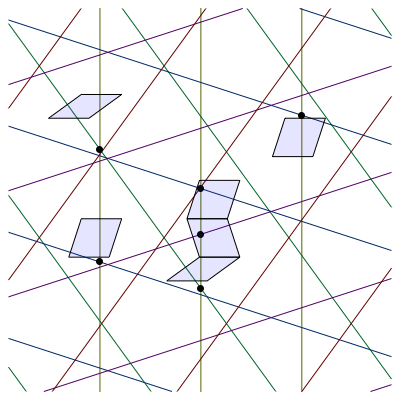The grid lines capture the incidence relationships between the tiles: if two points of intersection, Q1 and Q2, lie on the same grid line, and no other intersections come between them, then the corresponding tiles share an edge. However, there is no fixed relationship between the point Q and the location of the tile, because the lattice square is free to sit within the hypercube centred at Q in various ways in (n–2) dimensions.If we project all the lattice squares selected by this process, they fit together nicely: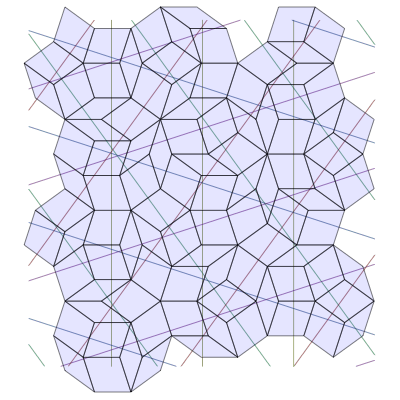We’ll now derive an explicit formula for the points Q where our families of lines intersect. If we choose the vector a in Rn for the centre of the coordinate system on our affine plane P, and use the vectors x and y divided by √(n/2) as an orthonormal basis, then the formula for the intersection of P with the hyperplane xk+1 = j+(1/2), where j is any integer, and k=0, …, n–1, will be:

bj,k + x cos(kπ/n) + y sin(kπ/n) = 0

Here x and y are coordinates on P with respect to our chosen basis, and we’ve defined the abbreviation:

bj,k = (–1)k (ak+1j – 1/2) √(n/2) .

The kth family of lines will be orthogonal to the vector (cos(kπ/n), sin(kπ/n)), and the successive lines in a given family will be separated by a distance of √(n/2).

The point where the (j,k) line intersects the (r,s) line will have coordinates:

x = [bj,k sin(sπ/n) – br,s sin(kπ/n)] / sin((ks)π/n)
y = [– bj,k cos(sπ/n) + br,s cos(kπ/n)] / sin((ks)π/n)If we wish to avoid degenerate points, where more than two lines intersect, we need to be careful with our choice of the offset vector a. For example, if we choose a=(1/2, 1/2, …, 1/2) we have bj,k = (–1)k j √(n/2). As well as the fact that all of the j=0 lines for the various k values will intersect at the origin, it’s not hard to show that, for any integer m, the intersections of the (j,k) line with the (0, (k+m) mod n) line and with the (–j, (k+2m) mod n) line will also be identical. Translated into geometric terms, this is just a matter of one line passing through the origin bisecting the angle where two lines with equal offsets from the origin meet, as in the image on the left.

If we assume that the solution we found above for the intersection of the (j,k) and (r,s) lines also lies on the (u,v) line, we find that for a degenerate point we’d need to have:

bu,v sin((ks)π/n) + bj,k sin((sv)π/n) + br,s sin((vk)π/n) = 0

If none of the coordinates of a is an odd half-integer, then none of the b values here can be zero, and if we divide out a common factor of √(n/2), this becomes a matter of the b values being such that they can weight a combination of three sines of rational multiples of π so that they sum to zero. Given that k, s and v are distinct and all lie between 0 and n–1, it’s not too hard to choose a to make this impossible; in fact, a=(0, 0, …, 0) will suffice, since that restricts the b values themselves to odd half-integers, and there is never a choice of k, s and v that can make all three sines rational.

In general, avoiding odd half-integers for a and values contrived to solve the equation above will be enough to prevent degenerate points from arising in the tiling.

#### Exact rotational symmetries

We won’t analyse the statistical properties of these tilings: their quasi-periodicity and rotational quasi-symmetries. However, in some cases the tilings will have exact rotational symmetries about one point. In analysing these exact symmetries, there are three maps of Rn that are of interest to us:

The map C, where we cycle all the coordinates of Rn:

C : (x1, x2, …, xn) → (xn, x1, …, xn–1)

the map D, where we cycle the coordinates and then change the sign of the new first coordinate:

D : (x1, x2, …, xn) → (–xn, x1, …, xn–1)

and the map E = –C, where we cycle the coordinates and change the sign of every coordinate:

E : (x1, x2, …, xn) → (–xn, –x1, …, –xn–1)

All three are symmetries of the hypercubic lattice: they preserve distances, and map all lattice points to other lattice points. The map C has order n, i.e. Cn is the identity map, and C has a 1-dimensional subspace of invariant vectors: C(a, a, …, a) = (a, a, …, a). The map D has order 2n, and no invariant vectors. The map E has order n if n is even, and order 2n if n is odd. If n is even, E has a 1-dimensional subspace of invariant vectors: E(a, –a, …, a, –a) = (a, –a, …, a, –a); if n is odd, E has no invariant vectors.

If n is odd and we apply C to the vectors x and y that span the subspace parallel to the plane P, we find:

C x = (cos((n–1)π/n), 1, –cos(π/n), cos(2π/n) …, (–1)(n–2)cos((n–2)π/n)) = –cos(π/n) x – sin(π/n) y
C y = (sin((n–1)π/n), 0, –sin(π/n), sin(2π/n) …, (–1)(n–2)sin((n–2)π/n)) = sin(π/n) x – cos(π/n) y

So C acts on the subspace as a rotation by an angle of π/n followed by a negation, which is the same as a net rotation by (n+1)π/n. If n is even, the map D has the same effect:

D x = (cos((n–1)π/n), 1, –cos(π/n), cos(2π/n) …, (–1)(n–2)cos((n–2)π/n)) = –cos(π/n) x – sin(π/n) y
D y = (sin((n–1)π/n), 0, –sin(π/n), sin(2π/n) …, (–1)(n–2)sin((n–2)π/n)) = sin(π/n) x – cos(π/n) y

If n is even, the map C doesn’t preserve the subspace spanned by x and y, and if n is odd, the map D doesn’t preserve the subspace.

Since the map E is just –C, if n is even it also won’t preserve the subspace, but if n is odd it will, and it will undo the negation we mentioned C producing, leaving just a rotation by an angle of π/n.

By considering the orders of these maps and their effects on both the subspace parallel to our chosen plane P and the offset vector a that we’ve chosen for P, we can make sense of the degree of symmetry that we see in various tilings.

#### Odd n

n=5, a=(0,0,0,0,0) n=5, a=(1/5, 1/5, 1/5, 1/5, 1/5)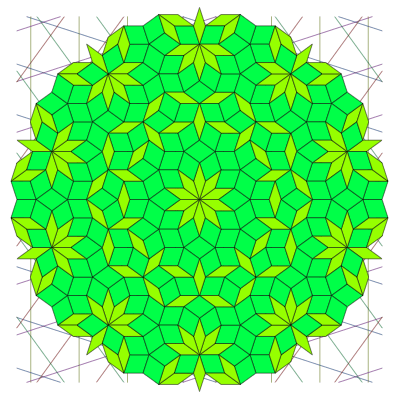In the image above left, we’ve used n=5, a=(0,0,0,0,0). The map E, with order 10, preserves the subspace, which in this case is equal to P. So the tiling has perfect 10-fold symmetry about the origin.

In the image above right, we’ve used n=5, a=(1/5, 1/5, 1/5, 1/5, 1/5). The map E doesn’t preserve a, so we don’t have 10-fold symmetry, but the map C, with order 5, does preserve a. So the tiling has perfect 5-fold symmetry about the origin.

The difference between the two cases shows up in the positioning of the five families of lines in P. In the a=(0,0,0,0,0) case (below left), each family of lines is positioned symmetrically with respect to the origin — that is, the origin falls precisely midway between two of the lines — so as well as being able to rotate one family into another, we can also rotate one family into the opposite of another. That gives us a 10-fold symmetry. But in the a=(1/5, 1/5, 1/5, 1/5, 1/5) case (below right), the lines that lie on either side of the origin are at different absolute distances from it, so they can’t be rotated into each other.

n=5, a=(0,0,0,0,0) n=5, a=(1/5, 1/5, 1/5, 1/5, 1/5)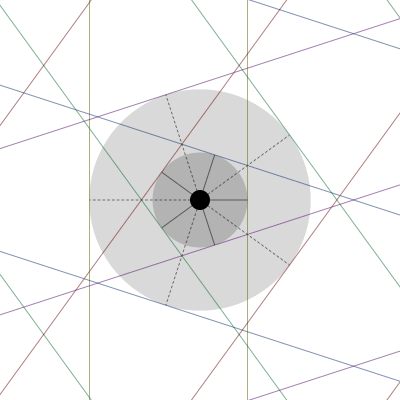#### Even n

n=6, a=(0,0,0,0,0,0) n=6, a=(1/6, 1/6, 1/6, 1/6, 1/6, 1/6)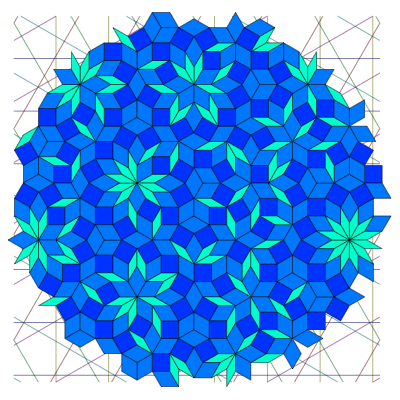In the image above left, we’ve used n=6, a=(0,0,0,0,0,0). The map D, with order 12, preserves the subspace, which in this case is equal to P. So the tiling has perfect 12-fold symmetry about the origin.

In the image above right, we’ve used n=6, a=(1/6, 1/6, 1/6, 1/6, 1/6, 1/6). The map D does not preserve a, so the tiling has no exact rotational symmetry about the origin.

What’s happening in the plane P? For the a=(0,0,0,0,0,0) case (below left), we have six families of lines, all positioned symmetrically about the origin. We can rotate any family of lines into any other family, or into its opposite, giving us a 12-fold symmetry. But why don’t we have a 6-fold symmetry in the a=(1/6, 1/6, 1/6, 1/6, 1/6, 1/6) case (below right), where we have six families of lines with the same set of distances from the origin? Because the set of lines at a given distance are not parallel to the sides of a regular hexagon; the sides of a hexagon come in three parallel pairs, so our construction can only give rise to hexagonal symmetry via n=3, rather than n=6.

n=6, a=(0,0,0,0,0,0) n=6, a=(1/6, 1/6, 1/6, 1/6, 1/6, 1/6)### Higher-dimensional tilings

Instead of projecting onto a plane, we can project onto subspaces of three or more dimensions. The most interesting case involves choosing a three-dimensional subspace of R6 such that the projections of the six coordinate axes and their opposites give the twelve vertices of an icosahedron. The resulting quasicrystal has an icosahedral symmetry that is impossible in a normal, periodic crystal, just as the five-fold symmetry of the Penrose tiling is impossible in a periodic two-dimensional tiling.

To choose a suitable three-dimensional subspace of R6, we pick any regular icosahedron in R3 and locate the six axes that join its twelve vertices in pairs. We choose a unit vector that lies on each of these axes. If uj, j=1, …, 6, are the six unit vectors, and uji their coordinates in an orthonormal basis of R3, then the same uji give us, for i=1,2,3, the coordinates in R6 of three vectors which comprise an orthogonal basis of a three-dimensional subspace, P, such that the coordinate axes project onto P along the six icosahedral axes we started with.

How do we know that this procedure will give us an orthogonal basis? It is a result in group representation theory  that if you take any linear operator, S0, on a vector space V, and an irreducible representation R on V of a finite group G, then if you sum the conjugation of S0 by R(g) for all g in G:

S = ΣgG R(g) S0 R(g)–1

the sum S will be a multiple of the identity operator I. Take S0 to be the projection onto the axis through one vertex pair, say S0ik = u1iu1k, then choose G to be the 60-element group of the rotational symmetries of the icosahedron, with R the fundamental representation of this group as rotations in R3. Conjugation with R(g) will simply transform S0 into a projection onto the various axes through the vertices, with each of the six axes appearing ten times. So S will be ten times the sum of projectors onto all six vertex pairs:

Sik = 10 Σj=1, …, 6 ujiujk

That the matrix for S is a multiple of the identity matrix then implies that the three 6-dimensional vectors with coordinates uji are orthogonal to each other. Note that we could also use this approach to find projections from spaces of dimension 10, 15, or 30 by choosing a face or edge centre of the icosahedron, or a generic point, as our starting vector. (The icosahedron has 20 faces and 30 edges, whose centres lie on 10 and 15 axes respectively. A generic point will have a 60-point / 30-axis orbit under the symmetry group.)

Having specified P, we then have six families of planes where P intersects the hyperplanes xi = odd-half-integer. These planes will be orthogonal to the projections of the coordinate axes, and since those projections are aligned with the vertices of an icosahedron, the orthogonal planes will be parallel to the faces of the icosahedron’s dual, a dodecahedron. Wherever three of these planes intersect, we have a point where we can place the centre of a 6-cube such that it will simultaneously enclose eight lattice points in a cubic configuration. We project those cubes onto P to find the rhomboid-shaped tiles of the quasiperiodic tiling.

The animation below shows a piece of a quasicrystal with icosahedral symmetry; rather than drawing the rhomboids we have drawn small spheres where the centre of each tile would be, with a colour determined by the shape of the tile.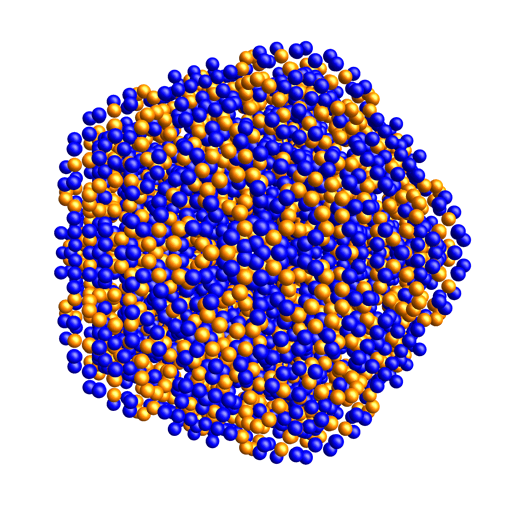We’ve chosen to have the subspace P pass through the origin, in order to give perfect icosahedral symmetry around one point, but there’s a small hitch that arises from doing this; it leads to there being some points in P where a 6-cube encloses more than the eight lattice points of a 3-cube, and takes in either a full 4-cube or a full 5-cube.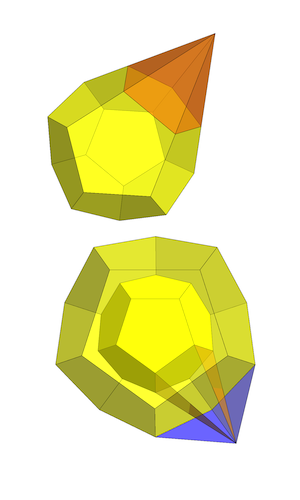We won’t calculate the coordinates of these degenerate points, but the existence of one set isn’t hard to picture. We’ve already mentioned that the families of planes in P are parallel to the faces of a regular dodecahedron. The image on the top left shows how five such planes, if equidistant from the origin, can meet at a single point, through the extension of five faces of the dodecahedron into the sides of a pentagonal pyramid.

The image on the bottom left shows how four planes which come from two different-sized dodecahedra with parallel faces can intersect in a single point. Note that the ratio of the sizes doesn’t need to take on any special value; the four planes will still meet at one point, regardless.

This situation could be finessed in various ways, but for the animation above we’ve just projected down multiple 3-cubes from within the higher dimensional cubes and drawn a sphere at the centre of each. So as the price of its perfect symmetry, this crystal has some “crowded” sites. The problem vanishes if P is given a generic irrational offset from the origin in R6.

### Other lattices

We can generalise the method we’ve described to apply to other lattices in Rn besides the hypercubic lattice . First, we consider the Voronoi cells associated with each point p in the lattice: these are the subsets of Rn consisting of points that are either closer to p than to any other point in the lattice, or equidistant from p and some other lattice point. Midway between two neighbouring lattice points p and q, the Voronoi cells of p and q will intersect along a part of their boundaries.

The Voronoi cells of a lattice in Rn are all congruent n-dimensional polytopes that pack together to fill Rn. Dual to this packing we can also consider the Delaunay cells, which are the convex hulls of the sets of lattice points whose Voronoi cells share a vertex in common; we say the Delaunay cell is dual to that Voronoi cell vertex. Unlike the Voronoi cells, the Delaunay cells need not all be congruent. (Note that “Delaunay” is sometimes spelled “Delone” .)

We can extend the notion of the Delaunay cell dual to the vertex of a Voronoi cell by noting that for each integer m=0, …, n–1, the Voronoi cells will have various “m-boundaries” of dimension m (i.e. vertices, edges, 2-faces, …, (n–1)-faces) which will be shared by certain sets of adjoining Voronoi cells. We define the corresponding “dual (nm)-boundaries” of the Delaunay cells to be the convex hulls of those lattice points whose Voronoi cells intersect at the m-boundaries. For example, in Z3 the Voronoi cells are cubes of unit side length centred on each lattice point. Each face of one of these cubes is a Voronoi 2-boundary; dual to it is a Delaunay 1-boundary, or edge, consisting of a line segment joining the two lattice points whose Voronoi cells share the face in question.

If our projection space P has dimension m, we can choose to project selected m-boundaries of either the Voronoi cells or the Delaunay cells associated with the lattice. If we want to project m-boundaries of the Voronoi cells, we think of P as being divided into regions that intersect different Delaunay cells. The corners of these regions, where multiple Delaunay cells intersect P, will actually be the points of intersection of P with certain (nm)-boundaries of the Delaunay cells; we then project the Voronoi cell m-boundaries dual to those Delaunay cell (nm)-boundaries.

If instead we want to project the m-boundaries of the Delaunay cells, we reverse the roles of Delaunay and Voronoi cells in this procedure, and project the Delaunay cell m-boundaries dual to those Voronoi cell (nm)-boundaries that intersect P.

For a hypercubic lattice, the Voronoi cells and Delaunay cells are both hypercubes packed into Rn in identical ways, so there is no real distinction between the two choices.

### The lattice An

One of the simplest examples where the Voronoi and Delaunay cells are different is the family of lattices known as An. The n-dimensional lattice An consists of those points of the (n+1)-dimensional hypercubic lattice Zn+1 that lie in the subspace satisfying x1 + x2 + … + xn+1=0. Part of the lattice A2 embedded in Z3 is shown below; the purple spheres are in A2.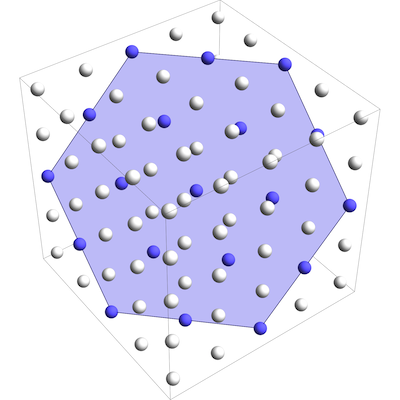Rather than choosing a basis for this subspace and working in n dimensions, it will make our calculations simpler if we keep working in (n+1) dimensions. We just have to remember that we’re imposing the condition x1 + x2 + … + xn+1=0 on everything.

The origin of Rn+1 lies in An, and its nearest neighbours in An will be all the points that lie a distance of √2 away and whose coordinates still sum to zero. These will take the form (1, –1, 0, 0, …), (1, 0, –1, 0, …), etc., where we choose any two of the n+1 coordinates to be non-zero, and then make either the first one +1 and the second one –1 or vice versa. That means there are a total of n(n+1) nearest neighbours to every lattice point. Every Voronoi cell in An will have a hyperface, or (n–1)-boundary, that it shares with each of its nearest neighbours. These hyperfaces will lie in the hyperplanes that orthogonally bisect lines joining the lattice point to its neighbours. The Voronoi cells in A2 are the hexagons in the image below; the Delaunay cells are the dashed triangles.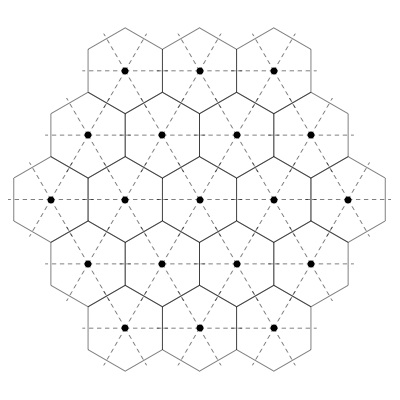What can we say about the vertices of the Voronoi cell around the origin? Each vertex will belong to at least n hyperfaces with linearly independent normals, since each hyperface imposes a single linear equation, and we need n independent equations to pin down a single point in our n-dimensional subpace. This means the vertex’s dot product with (at least) n distinct vectors with 1 and –1 as their only non-zero coordinates must equal 1 (the norm of such vectors is √2, and the bisected distance to each nearest neighbour is 1/√2, so in the dot product these factors cancel to give 1). Also, to lie within the bounds of the Voronoi cell, the vertex’s dot product with any vector of that form cannot exceed 1.

Consider the point v1=(n/(n+1), –1/(n+1), –1/(n+1), …, –1/(n+1)). Clearly this lies in our subpace, since the coordinates sum to zero. If we consider the n neighbours of the origin (1, –1, 0, 0, …), (1, 0, –1, 0, …), and so on, each one will have a dot product with v1 of exactly 1. And if we take the dot product of v1 with any vector with 1 and –1 as its only non-zero coordinates, the result can only be 0, 1, or –1. So v1 will be a vertex of the Voronoi cell, as will all n+1 similar vectors where we choose different coordinates to take the value n/(n+1).

To generalise this, suppose v=(1–s, 1–s, …, –s, –s, …), where we have q coordinates of value 1–s and (n+1–q) coordinates of value –s; we require q to be at least 1 and not more than n. For the sum of the coordinates to be zero, we need q(1–s)–(n+1–q)s=0, or s=q/(n+1). There will be q(n+1–q) different neighbours of the origin (a number that’s always at least n) which give us a dot product with v of 1, and it’s clear that no difference of two coordinates can exceed 1. There are (n+1)!/[q!(n+1–q)!] ways of choosing the q coordinates that are set to 1–s, so the total number of vertices of this kind works out to 2n+1–2; this is just the usual sum of coefficients in a binomial expansion, with the q=0 and q=n+1 terms omitted.

If we try to vary this construction by introducing a third coordinate value, it becomes impossible to obtain the dot product of 1 with a linearly independent set of n hyperface normals. So these are all the vertices of the Voronoi cell around the origin.

The count of 2n+1–2 should ring a bell; this is the number of vertices in an (n+1)-dimensional hypercube, minus two! Why minus two? If we project all the vertices in a unit-side-length (n+1)-cube centred on the origin into our subspace, exactly two vertices, (1/2, 1/2, 1/2, …, 1/2) and (–1/2, –1/2, –1/2, …, –1/2), will be projected onto the origin. The remaining 2n+1–2 points will be precisely the vertices of the Voronoi cell of the origin in An. If we take any vertex (±1/2, ±1/2, ±1/2, …, ±1/2) of the (n+1)-cube with q positive coordinates, and project it into our subspace (which amounts to subtracting off its projection onto the unit vector (1, 1, 1, … 1) / √[n+1], or equivalently summing the coordinates, dividing the sum by n+1, then subtracting the result from each coordinate), we get one of the An Voronoi vertices previously described, with q coordinates of the form 1–q/(n+1).

We can enumerate the m-boundaries of the An Voronoi cell simply by excluding the m-boundaries of the (n+1)-cube that contain the two vertices that project to the origin; we exclude those m-boundaries because they project into the interior of the An Voronoi cell. The full count of m-boundaries of an (n+1)-cube is 2n+1–m (n+1)!/[m!(n+1–m)!] (derived here). Omitting those that contain the two excluded vertices, we get:

Number of m-boundaries for the An Voronoi cell = (2n+1–m–2) (n+1)!/[m!(n+1–m)!]

Now, as we’ve noted, the vertices of the Voronoi cells each have associated with them a number q, which for the Voronoi cell around the origin is a count of the number of coordinates whose value is 1–s (where s=q/(n+1)). This is also the number of coordinates whose value is +1/2 in the (n+1)-cube vertex that we projected down to get the Voronoi cell vertex. This vertex will be shared between the Voronoi cell at the origin and those of q(n+1–q) nearest neighbours, because we have q choices for where we put the 1 in a nearest-neighbour vector so it lines up with one of the coordinates whose value is 1–s, and (n+1–q) choices for putting a –1 that lines up with one of the coordinates whose value is –s, in order to get the required dot product of 1. So the vertex will lie at the intersection of q(n+1–q) hyperfaces, each of which will be shared with a nearest-neighbour lattice point.

However, a Voronoi cell vertex can also be shared between two lattice points that aren’t nearest neighbours; in that case the Voronoi cells of the two lattice points don’t have a shared hyperface. For example, the vertex v=(1/2, 1/2, –1/2, –1/2) of the Voronoi cell of the origin in A3 will be shared by the four nearest-neighbour lattice points (1,0,–1,0), (1,0,0,–1), (0,1,–1,0) and (0,1,0,–1), and also by the lattice point L=2v=(1,1,–1,–1). The easiest way to see this is to note that –v is also a vertex of the Voronoi cell of the origin, and so by translation symmetry Lv=v must be a vertex of the Voronoi cell of L.

In general, suppose v=(1–s, 1–s, …, –s, –s, …), with q coordinates of the form 1–s. We can count the total number of lattice points on whose Voronoi cells it lies by looking for lattice points L such that vL is a vertex of the Voronoi cell of the origin. If L contains an equal number of coordinates with value 1 and value –1, with all those of value 1 lined up with a 1–s in v and all those of value –1 lined up with a –s in v, subtracting L from v will simply exchange a certain number of 1–s and –s entries, so it will continue to be a vertex of the Voronoi cell of the origin. For each integer 0≤k≤max(q, n+1–q), there will be q!/[k!(qk)!] ways to choose k coordinates from the q with value 1–s, and (n+1–q)!/[k!(n+1–qk)!] ways to choose k coordinates from the n+1–q with value –s, so we need to sum the product of these expressions over k to get the total:

Σk q! (n+1–q)! / [(k!)2 (qk)! (n+1–qk)!]
= (n+1)! / [q! (n+1–q)!]

The Delaunay cell dual to each vertex of the Voronoi cell is the convex hull of the lattice points whose Voronoi cells intersect at that vertex, so we can see that the Delaunay cell will have a different shape depending on the q value for the vertex; in fact, the number of lattice points in the Delaunay cell dual to a vertex with a given q value turns out to be equal to the number of Voronoi cell vertices with that q value. (This is not a coincidence, as we’ll see shortly.) For example, in A3, there are 4, 6 and 4 vertices for q=1,2,3 respectively, and the total number of lattice points in the Delaunay cells dual to those vertices are 4, 6 and 4. The Delaunay cells are tetrahedra and octahedra; there are eight tetrahedral Delaunay cells with the origin as a vertex, and six octahedra. In the image below, we only show the Delaunay cell faces that intersect the origin. The Voronoi cell for the origin is the blue polyhedron with 12 rhombic faces in the centre.Now, the vertices of the Delaunay cells that include the origin all have integer coordinates that are either 0, 1 or –1. This means that they are a subset of the vertices of the 2n+1 unit hypercubes in Zn+1 that have the origin as one of their vertices. More precisely, it turns out that each particular one of the 2n+1–2 Delaunay cells that include the origin (one dual to each vertex of the origin’s Voronoi cell) is simply the intersection with the subspace S of one of those hypercubes! Each vertex v of the origin’s Voronoi cell is found by projecting a point of the form c=(±1/2, ±1/2, ±1/2, …, ±1/2) onto S, and v will take the form 1–s where c is 1/2, and –s where c is –1/2. If we take the unit hypercube centred on c, its vertices h will all be 0 or 1 where c is 1/2, and 0 or –1 where c is –1/2. So, those hypercube vertices h whose coordinates sum to zero will be precisely those lattice points of An whose Voronoi cell includes v, which is, by definition, the set of vertices of the Delaunay cell dual to the Voronoi-cell vertex v.

This shows us exactly where the “coincidence” between the count of Voronoi vertices with a given q value and the number of lattice points in the Delaunay cell dual to one of those Voronoi vertices comes from. The sum of the coordinates of one of our points c has a simple relationship to the q value: it’s just q–(n+1)/2. If we translate a unit hypercube away from the origin and centre it on c, the number of vertices of that translated hypercube whose coordinates sum to zero, and hence lie in S, will equal the number of vertices in the untranslated unit hypercube whose coordinates sum to the opposite value, (n+1)/2–q. But by symmetry (of the hypercube, or of the binomial formula that counts the vertices with a given q value) this is exactly the same as the number of vertices in the untranslated hypercube whose coordinates sum to q–(n+1)/2.

Each m-boundary of the Voronoi cell will contain 2m vertices, being a projection of an m-boundary of an (n+1)-cube. For this set of vertices, n+1–m of their coordinates will have a fixed form (always being either 1–s or –s, though the actual value of s=q/(n+1) will vary), and the other m will take all 2m possible choices between the two forms 1–s and –s. As a result, the q value of the vertices in the m-boundary will range from some mimimum q0, that must be at least 1 and at most nm, up to q0+m.

The Delaunay cell (nm)-boundary dual to a given Voronoi cell m-boundary will be the convex hull of all the lattice points whose Voronoi cells intersect to give the m-boundary. This will be the intersection with the subspace S of the Delaunay (n+1–m)-boundary in Zn+1 dual to the Voronoi m-boundary in Zn+1 that projects to our chosen Voronoi m-boundary in An. More directly, the set of lattice points comprising the vertices of the Delaunay (nm)-boundary will be the intersection of the sets of lattice points dual to each vertex in the Voronoi m-boundary. Because of the way lattice points sharing a vertex are constructed, this set will be determined solely by the part of the vertex coordinates that is fixed across the whole Voronoi m-boundary, and its size will be given by the sum, where k ranges from 0 to max(q0, n+1–mq0):

Σk q0! (n+1–mq0)! / [(k!)2 (q0k)! (n+1–mq0k)!]
= (n+1–m)! / [q0! (n+1–mq0)!]

For example, suppose n=4, m=2. The Voronoi cell will have (23–2) 5!/[2!3!] = 60 2-boundaries, all with four vertices. Each 2-boundary will have a q0 ranging from 1 to 2, yielding lattice point counts of 3 and 3 respectively. So the dual 2-boundaries of the Delaunay cells will be the convex hulls of three lattice points; in other words, they will be triangles.

In fact, for any value of n, the Delaunay 2-boundaries (which are dual to Voronoi (n–2)-boundaries) will be triangles; for m=n–2, we will always have q0 ranging from 1 to 2, yielding lattice point counts of 3 and 3.

If we have a plane P that lies in An, we can choose to project either the triangular 2-boundaries of the Delaunay cells or the quadrilateral 2-boundaries of the Voronoi cells onto P. In the first case, we project those Delaunay 2-boundaries dual to Voronoi (n–2)-boundaries that intersect P; in the second case, we project those Voronoi 2-boundaries dual to Delaunay (n–2)-boundaries that intersect P.

#### Calculating the projection of triangular Delaunay 2-boundaries onto a plane

Let’s look at the case where we project the triangular 2-boundaries of the Delaunay cells in more detail. We’ve described the mathematics on a conceptual level, but how can we perform these calculations in practice?

For the hypercubic lattice, we made use of the fact that all the Voronoi cell (n–1)-boundaries lay on hyperplanes of the form xi = j + 1/2, where i indexed the n coordinates and j was any integer. These n families of hyperplanes intersected our plane P as n families of parallel lines, and the intersections between the lines from different families gave us the intersections of the Voronoi (n–2)-boundaries with P.

To find the points where the Voronoi (n–2)-boundaries in An intersect P, we note that once again the Voronoi (n–1)-boundaries will lie on certain hyperplanes. If we take n(n+1)/2 vectors such as (1,–1,0,0,…) which lie on the rays joining the lattice point at the origin with its nearest neighbours, all the Voronoi (n–1)-boundaries will lie on hyperplanes where the dot product with one of these vectors is an integer. Unlike the case with the hypercubic lattice, however, portions of these hyperplanes will not lie on the boundaries of the Voronoi cells. A glance at the hexagonal Voronoi cells in A2 should make this clear: unlike the case with a square grid, extending the border of any cell beyond its endpoints (the dashed lines in the image below) gives you a line that travels into the interior of the neighbouring cell rather than consisting solely of cell borders. So we need to be prepared to throw out some “false positives”: some points on P where the lines from these hyperplanes intersect but which do not lie on the boundary of any Voronoi cell.Another subtlety we need to account for is that every point where two of the lines intersect will also have a third line passing through it; again the case of A2, where the Voronoi cells’ corners all lie on three lines, makes this clear. For the hypercubic lattice, we looked at every intersection between every pair of hyperplanes chosen from n different families; for the An lattice, looking at every pairing between our n(n+1)/2 families of hyperplanes would be overkill. Instead, we note that every (n–2)-boundary lies on the intersection of three hyperplanes that bear a particular relationship to each other, and we can single out the intersection by referring to just two of the hyperplanes. What’s more, we can enumerate a particular subset of suitable pairs to use, as follows.

Recall that the (n–2)-boundaries of the Voronoi cell of the origin in An are projections of (n–2)-boundaries of the unit hypercube centred on the origin in Zn+1; associated with each (n–2)-cube in Zn is a choice of three coordinates from the total of n+1 which defines the 3-space orthogonal to that (n–2)-cube. There are (n+1)n(n–1)/6 such choices. Given a specific choice of three coordinates, i1 < i2 < i3, we pick the pair of vectors n1 = ei1ei2 and n2 = ei1ei3, where the ei are standard basis vectors in Rn+1. For the Voronoi cell of the origin, the vertices of each particular (n–2)-boundary associated with this choice of i1, i2, and i3 will be 2n–2 points that take all possible choices for the forms 1–s and –s for the remaining n–2 coordinates, while having one particular choice of those forms for the i1, i2, and i3 coordinates. The details of the six possibilities are as follows:

The six Voronoi (n–2)-boundaries for a given coordinate triple i1, i2, i3
xi1xi2xi3 n1 · x
n1 = ei1ei2
n2 · x
n2 = ei1ei3
Two vectors that give a dot product of 1
nAnB
1–s1–ss01n2n2n1
1–ss1–s10n1n1n2
s1–s1–s–1–1n1n2
1–sss11n1n2
s1–ss–10n1n2n1
ss1–s0–1n2n1n2

Why only six possibilities, not eight? We need to exclude vertices that are all 1–s or all –s, because the requirement for the coordinates to sum to zero would force any such point to lie at the origin, not on the cell’s boundary.

The dot products in this table are for the Voronoi cell of the origin, but displacing the cell away from the origin can only add integers to the result. So we look for candidate points to be intersections of P with Voronoi (n–2)-boundaries in general by examining the intersections of pairs of lines on P for which n1 and n2 respectively have integer dot products.

Now, if a point has an integer dot product with both n1 and n2, it will also have an integer dot product with n3 = n2n1 = ei2ei3. But we don’t need to look for intersections between the lines associated with n3 and those associated with n1 or n2, because we know that they will already be covered by the n1, n2 intersections. The (n+1)n(n–1)/6 pairs of hyperplane families that we generate from the (n+1)n(n–1)/6 choices of coordinate triples covers everything.

Given a point that lies on the intersection of our plane P with one of the pairs of hyperplanes described above, how can we tell whether it’s a “false positive” or a point on a Voronoi (n–2)-boundary? And if it does lie on a Voronoi (n–2)-boundary, what are the coordinates of the three lattice points whose Voronoi cells share that (n–2)-boundary?

For any given coordinate triple and associated pair of hyperplane families, and for each of the six possibilities in our table above, we can construct a set of n+1 linearly independent vectors as follows. First, we include the two vectors from the last two columns in our table, both of which are guaranteed to have a dot product of exactly 1 with every point in our chosen (n–2)-boundary of the Voronoi cell of the origin. Call these {nA, nB}. Then we add n–2 vectors that all have a coordinate of 1 for the first of i1, i2, or i3 that takes the form 1–s, 0 for the other coordinates in the triple, and –1 in exactly one of the n–2 remaining coordinates. Call these vectors {p1, … pn–2}. Each of the pj will have a dot product of 1 with exactly half the vertices in our chosen (n–2)-boundary of the Voronoi cell of the origin: those who have a –s in the same place as its –1; with the other vertices, the same vector will have a dot product of 0. What’s more, for any point on the chosen (n–2)-boundary the dot product with all the pj will lie between 0 and 1. Finally, we throw in the diagonal vector d = (1,1,…,1), which is orthogonal to everything in the lattice.

Given some candidate point x that we found at the intersection of two of our lines on P, we form its dot product with everything in the appropriate set of n+1 vectors. We have six such sets, so to start with we just use the first one. Suppose there is a lattice point L such that xL lies on a (n–2)-boundary of the Voronoi cell of the origin, where the coordinate triple corresponding to the pair of intersecting lines on P, and our choice from the six possibilities, together pin down the particular (n–2)-boundary. Then we have (xL) · nA and (xL) · nB equal to 1, so L · nA, B = x · nA, B – 1.

We also have 0 ≤ (xL) · pj ≤ 1, so L · pjx · pjL · pj+1. But L · pj is an integer (since all the coordinates of both vectors are integers), and that integer must be floor(x · pj), the largest integer not greater than x · pj.

The dot product of L with d is zero, of course. Having specified the dot product of L with n+1 linearly independent vectors, it’s a straightforward calculation to use the inverse of the matrix formed from the coordinates of those vectors to find the coordinates of L itself.

But what if the first of our six possible choices was the wrong one? Or what if the point x isn’t really on the boundary of any Voronoi cell? It turns out that in either case, we get non-integer coordinates for our putative L, so we know the result can’t be right. What’s more, for any given point that is on a Voronoi (n–2)-boundary, either the first three of the possibilities listed in our table will all give valid lattice points L, or the last three will; the crucial difference here is whether the form 1–s appears two times or just once. So we only need to check one case for each of those two classes in order to classify x, and having found the right class we can go on to find the other two lattice points. In fact, given one lattice point L on whose Voronoi (n–2)-boundary x lies, it will also lie on the (n–2)-boundaries of L+nA and L+nB, giving us the complete dual triangle immediately.

The images below show projections of the triangular Delaunay 2-boundaries onto planes in A4, A6, A8 and A10. These tilings are known as “Tübingen triangular tilings” . For even n, we can use the planes previously described for projections onto the hypercubic lattice one dimension higher. The projections of the coordinates axes of Rn+1and their opposites form a 2(n+1)-pointed star in which positive and negative axes alternate; the directions of the edges of the Delaunay 2-boundaries, which are always a difference between coordinate vectors, project down to a 2(n+1)-pointed star that bisects the angles of the coordinate-axes star, and so the tile edges lie on a set of n+1 distinct rays.

Delaunay 2-boundaries from A4 Delaunay 2-boundaries from A6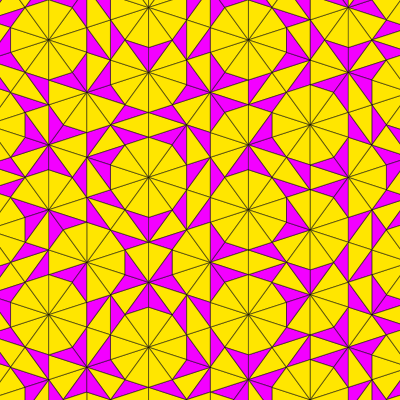If we wish to use an odd value for n we need to choose a different plane, because the plane we project onto for the hypercubic lattice doesn’t lie in the subspace that contains An. A suitable choice is the plane spanned by:

x = (1, cos(2π/(n+1)), cos(4π/(n+1)), …, cos(2nπ/(n+1)))
y = (0, sin(2π/(n+1)), sin(4π/(n+1)), …, sin(2nπ/(n+1)))

The images below show projections of the Delaunay 2-boundaries onto this plane in A5, A7, A9 and A11. [Note that the A5 projection is exactly periodic, but the precise pattern will vary depending on the offset chosen for the affine plane.] The projections of the coordinate axes of Rn+1and their opposites form an (n+1)-pointed star, with the projections of the negative axes overlapping with the those of the positive axes. The projections of the edges of the Delaunay 2-boundaries form a 2(n+1)-pointed star, comprising both the coordinate-axes star and bisectors of its angles, and so the tile edges again lie on n+1 distinct rays.

Delaunay 2-boundaries from A5 Delaunay 2-boundaries from A7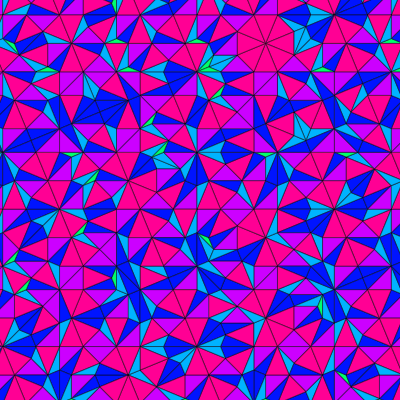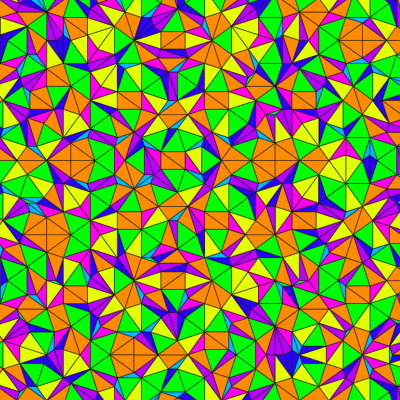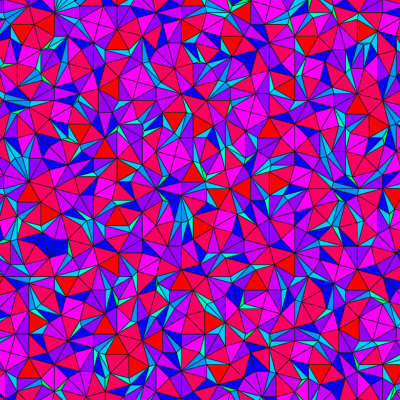#### Projecting the Voronoi 2-boundaries

What kind of tiling do we get from the An lattice if we choose to project the Voronoi 2-boundaries, rather than the Delaunay 2-boundaries, onto our plane P? The Voronoi 2-boundaries in An are rhombuses found by projecting 2-faces of the hypercubic Voronoi cells of Zn+1 onto our subspace S of points whose coordinates sum to zero. So long as the plane P lies in S, projecting those 2-boundaries from An down to P will be the same as projecting the original hypercube 2-faces from Zn+1 down to P. For even n — and hence odd n+1 — and an offset of zero, the plane P we used for the original deBruijn method does lie in S, and it turns out that the tilings produced from An will be identical to those produced from Zn+1.

Given that every Voronoi 2-boundary, F, in An is a projection onto S of some Voronoi 2-face, F*, in Zn+1, that claim probably isn’t too surprising, but to see that the tilings really are exactly the same, we need to show that the detailed selection process, not just the set of available tiles, is identical. This follows from the fact that we select Voronoi 2-boundaries in An to project on the condition that their dual Delaunay (n–2)-boundary intersects P. Each Delaunay (n–2)-boundary, D, in An is itself the intersection of the subspace S with a Delaunay (n–1)-face, D*, in Zn+1, which in turn is dual to a 2-face, F*, in Zn+1 whose projection onto S, F, is the dual in An of D. So it makes no difference whether the duality operation is performed in Zn+1 or An, and the criterion for selecting tiles works out identically, regardless.

``` rhombic                                    F                                         F*
tiles      project onto P              Voronoi            project onto S         Voronoi
on P ←·······················  2-boundaries in An ←······················· 2-faces in Zn+1
↑                                         ↑
|                                         |
|                                         |
| find dual                               | find dual
|                                         |
|                                         |
selection                                   ↓                                         ↓
points                                  Delaunay                                  Delaunay
on P ←······················· (n–2)-boundaries in An ←····················· (n–1)-faces in Zn+1
intersect with P                D               intersect with S          D*

```

For odd n, we can’t use the original choice of P since it doesn’t lie in S, but if we use the same plane as for the projection of the Delaunay 2-boundaries for odd n, the result will be a rhombic tiling with half the order of rotational symmetry as the tiling obtained from the hypercube projection.

### References

 N.G. de Bruijn, “Algebraic theory of Penrose’s nonperiodic tilings of the plane, I, II”, Nederl. Akad. Wetensch. Indag. Math. 43 (1981) 39–52, 53–66. (Available online as a PDF.) I learnt about this method from the documentation for Eugenio Durand’s program Quasitiler, which generates the same kind of tilings interactively via forms submitted to a server.

 S. Steinberg, Group Theory and Physics, Cambridge University Press, 1994. Proposition 3.1, section 2.3.

 Peter Kramer, “Dual Canonical Projections”.

 Wikipedia article on the mathematician Boris Delaunay.Applets Gallery / deBruijn (Technical Notes) / created Monday, 13 October 2008 / revised Wednesday, 24 December 2008